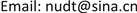1. 引言

2. 数值方法

∂ U ∂ t + ∂ E ∂ ξ + ∂ F ∂ η + ∂ G ∂ ζ = ∂ E v ∂ ξ + ∂ F v ∂ η + ∂ G v ∂ ζ (1)

3. 动导数计算方法

Dynamic damping derivative

C m = C m ( α ( t ) , α ˙ ( t ) , α ¨ ( t ) , ⋯ , q ( t ) , q ˙ ( t ) , q ¨ ( t ) , ⋯ ) (2)

C m q + C m α ˙ = 1 α m π ∫ t s t s + T c C m ( t ) cos k t d t (3)

4. 验证算例

5. 类X-51A支撑干扰计算

Comparison between the real shape and the experi-mental shap

6. 结论